The variation of acceleration of a particle executing SHM with displacement x is:

1.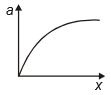2.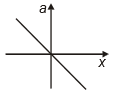3.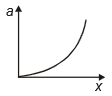4.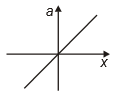Subtopic:  Simple Harmonic Motion |
To view explanation, please take trial in the course below.
NEET 2022 - Target Batch - Aryan Raj Singh
To view explanation, please take trial in the course below.
NEET 2022 - Target Batch - Aryan Raj Singh

Difficulty Level:

A particle is subjected to two simple harmonic motions in the same direction having equal amplitudes and equal frequency. If the resulting amplitude is equal to the amplitude of individual motions, the phase difference between them will be

1.  $\frac{\mathrm{\pi }}{3}$

2.  $\frac{2\mathrm{\pi }}{3}$

3.  $\frac{\mathrm{\pi }}{6}$

4.  $\frac{\mathrm{\pi }}{2}$

Subtopic:  Linear SHM |
To view explanation, please take trial in the course below.
NEET 2022 - Target Batch - Aryan Raj Singh
To view explanation, please take trial in the course below.
NEET 2022 - Target Batch - Aryan Raj Singh

Difficulty Level:

The motion of a particle varies with time according to the relation . Then

1.  The motion is oscillatory but not SHM

2.  The motion is SHM with an amplitude $a\sqrt{2}$

3.  The motion is SHM with an amplitude $\sqrt{2}$

4.  The motion is SHM with an amplitude a

Subtopic:  Simple Harmonic Motion |
To view explanation, please take trial in the course below.
NEET 2022 - Target Batch - Aryan Raj Singh
To view explanation, please take trial in the course below.
NEET 2022 - Target Batch - Aryan Raj Singh

Difficulty Level:

If a particle is executing SHM, with an amplitude A, the distance moved and the displacement of the body in a time equal to its time period are, respectively,

1.  2A, A

2.  4A, 0

3.  A, A

4.  0, 2A

Subtopic:  Linear SHM |
To view explanation, please take trial in the course below.
NEET 2022 - Target Batch - Aryan Raj Singh
To view explanation, please take trial in the course below.
NEET 2022 - Target Batch - Aryan Raj Singh

Difficulty Level:

The equations of the displacement of two particles making SHM are represented by y1 = a sin (ωt + φ) and y2 = a cos (ωt) respectively. The phase difference of the velocities of the two particles will be
1. π/2 + φ
2. -φ
3. φ
4. φ - π/2

Subtopic:  Linear SHM |
To view explanation, please take trial in the course below.
NEET 2022 - Target Batch - Aryan Raj Singh
To view explanation, please take trial in the course below.
NEET 2022 - Target Batch - Aryan Raj Singh

Difficulty Level:

The displacement of a particle executing SHM is given by y = 0.25 (sin 200t) cm. The maximum speed of the particles is:

1.  200 cm/sec

2.  100 cm/sec

3.  50 cm/sec

4.  0.25 cm/sec

Subtopic:  Simple Harmonic Motion |
To view explanation, please take trial in the course below.
NEET 2022 - Target Batch - Aryan Raj Singh
To view explanation, please take trial in the course below.
NEET 2022 - Target Batch - Aryan Raj Singh

Difficulty Level:

Which of the following figure represents damped harmonic motion?
(i)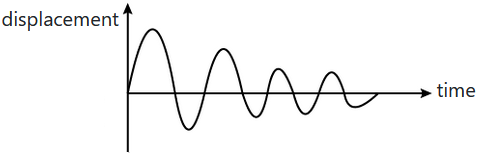(ii)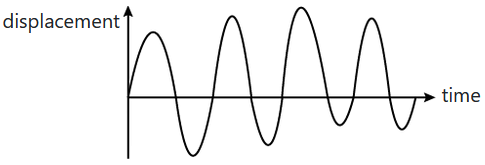(iii)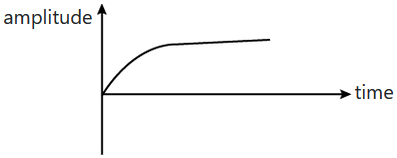(iv)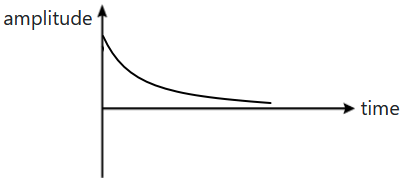1.  (i) and (ii)

2.  (iii) and (iv)

3.  (i), (ii), (iii), and (iv)

4.  (i) and  (iv)

Subtopic:  Damped Oscillations |
To view explanation, please take trial in the course below.
NEET 2022 - Target Batch - Aryan Raj Singh
To view explanation, please take trial in the course below.
NEET 2022 - Target Batch - Aryan Raj Singh

Difficulty Level:

A particle is executing SHM with an amplitude A and the time period T. If at t = 0, the particle is at origin (mean position), then the time instant when it covers a distance equal to 2.5A will be

1.

2.

3.

4.  $\frac{2\mathrm{T}}{3}$

Subtopic:  Linear SHM |
To view explanation, please take trial in the course below.
NEET 2022 - Target Batch - Aryan Raj Singh
To view explanation, please take trial in the course below.
NEET 2022 - Target Batch - Aryan Raj Singh

Difficulty Level:

The time period of a spring mass system at the surface of earth is 2 second. What will be the time period of this system on the moon where acceleration due to gravity is $\frac{1}{6}\mathrm{th}$ of the value of g on earth's surface?

1.

2.

3.

4.

Subtopic:  Spring mass system |
To view explanation, please take trial in the course below.
NEET 2022 - Target Batch - Aryan Raj Singh
To view explanation, please take trial in the course below.
NEET 2022 - Target Batch - Aryan Raj Singh

Difficulty Level:

A particle undergoes SHM with a time period of 2 seconds. In how much time will it travel from its mean position to a displacement equal to half of its amplitude?

(1) $\frac{1}{2}s$

(2) $\frac{1}{6}s$

(3) $\frac{1}{4}s$

(4) $\frac{1}{3}s$

Subtopic:  Simple Harmonic Motion |
To view explanation, please take trial in the course below.
NEET 2022 - Target Batch - Aryan Raj Singh
To view explanation, please take trial in the course below.
NEET 2022 - Target Batch - Aryan Raj Singh

Difficulty Level: